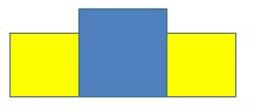# Dimensions 3159

The rectangle is 6 cm longer than the width. A square with a side equal to the length of the rectangle has an area 78 cm2 larger than the rectangle. Calculate the dimensions of the rectangle.

a =  13 cm
b =  7 cm

### Step-by-step explanation:Did you find an error or inaccuracy? Feel free to write us. Thank you!

Tips for related online calculators
Are you looking for help with calculating roots of a quadratic equation?
Do you have a linear equation or system of equations and looking for its solution? Or do you have a quadratic equation?

#### You need to know the following knowledge to solve this word math problem:

We encourage you to watch this tutorial video on this math problem: